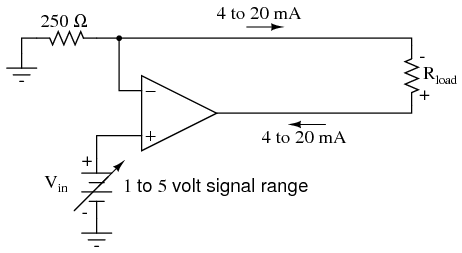# voltage current converter by op amp circuit

I am trying to convert 5V to a constant current sourcefor op amp I use AD822. for 250 ohm I use 180 ohm, so I should get a current of 28 mA. I verified when Rload = 0, 28 mA across negative terminal to ground.

But when I put Rload to circuit, 680 ohm, my current exceeds 28mA as Vcc increase.

Can anyone please point where might be the error?

• What does 'for 250 ohm I use 180 ohm' mean? Jun 9 '15 at 3:44
• What is Vcc in your circuit?? Jun 9 '15 at 4:35
• instead of 250 ohm I use 180 ohm. Vcc is at least +- 10V. Jun 9 '15 at 6:10
• The maximum current from 0 to 10v is approx 15mA. This tells me the connections are not as shown. Assuming your supply is connected to the opamp as required and the ground is at mid point between the +/-10v rails. Jun 9 '15 at 7:03
• sorry I meant I was increasing Vcc to see how current go. 18 +- Vcc should give me 28 mA when Rload = 680? but at 18V I get around 20 mA, and continue increasing as Vcc increase, and went negative at Vcc = +- 25V. ground is created as mid between two power supply. V+ is from arduino 5V. Jun 9 '15 at 7:23